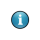On-line GuidesAll GuideseBook StoreiOS / AndroidLinux for BeginnersOffice ProductivityLinux InstallationLinux SecurityLinux UtilitiesLinux VirtualizationLinux KernelSystem/Network AdminProgrammingScripting LanguagesDevelopment ToolsWeb DevelopmentGUI Toolkits/DesktopDatabasesMail SystemsopenSolarisEclipse DocumentationTechotopia.comVirtuatopia.comAnswertopia.comHow To GuidesVirtualizationGeneral System AdminLinux SecurityLinux FilesystemsWeb ServersGraphics & DesktopPC HardwareWindowsProblem SolutionsPrivacy PolicyOpenOffice Calc 3.x Guide
Previous Page Home Next Page

## Order of calculation

Order of calculation refers to the sequence that numerical operations are performed. Division and multiplication are performed before addition or subtraction. There is a common tendency to expect calculations to be made from left to right as the equation would be read in English. Calc evaluations the entire formula, then based upon programming precedence breaks the formula down executing multiplication and division operations before other operations. Due to this fact when creating formulas you should test your formula to make sure that the correct result is being obtained. An example of order of calculation in operation follows.

Table 4 – Order of Calculation

 Left To Right Calculation Ordered Calculation 1+3*2+3 = 11 =1+3*2+3 result 10 1+3=4, then 4 X 2 = 8, then 8 + 3 = 11 3*2=6, then 1 + 6 + 3 = 10 Another possible intention could be: The program resolves the multiplication of 3 X 2 before dealing with the numbers being added. 1+3*2+3 = 20

If you intend for the result to be either of the two possible solutions on the left, the way to achieve these results would be to order the formula as:

 ((1+3) * 2)+3 = 11 (1+3) * (2+3) = 20Consider using parentheses grouping operations to avoid obtaining incorrect calculations, e.g. = B4+G12*C4/M12 becoming =((B4+G12)*C4)/M12.

OpenOffice Calc 3.x Guide
Previous Page Home Next PagePublished under the terms of the Creative Commons License Design by Interspire